## Indicators

•Cited by SciELO
•Access statistics

## Related links

•Similars in SciELO

## Print version ISSN 0035-001X

### Rev. mex. fis. vol.65 n.5 México Sep./Oct. 2019  Epub Apr 23, 2020

#### https://doi.org/10.31349/revmexfis.65.503

Research

in Gravitation, Mathematical Physics and Field Theory

The generalized exponential rational function method for Radhakrishnan-Kundu-Lakshmanan equation with β-conformable time derivative

a Department of Engineering Science, Kermanshah University of Technology, Kermanshah, Iran.

b Tecnológico Nacional de México/CENIDET. Interior Internado Palmira S/N, Col. Palmira, 62490, Cuernavaca, Morelos, México

c. Universidad Virtual CNCI, México. http://cnci.edu.mx/.

Abstract

In this paper, the generalized exponential rational function method is used to construct exact solutions of the β conformable-time Radhakrishnan-Kundu-Lakshmanan equation. This model governs soliton propagation dynamics through a polarization-preserving fiber. Fractional derivatives are described in the β-conformable sense. As a result, we get new form of solitary traveling wave solutions for this model including novel soliton, traveling waves and kink-type solutions with complex structures. Physical interpretations of some extracted solutions are also included through taking suitable values of parameters and derivative order in them. It is proved that this method is powerful, efficient, and can be fruitfully implemented to establish new solutions of nonlinear conformable-time partial differential equations applied in mathematical physics.

Keywords: Optical solutions; Exponential rational function method; Traveling waves; Exact solutions; Radhakrishnan-Kundu-Lakshmanan equation

PACS: 02.60.-x; 02.60.Cb; 02.30.Jr

1. Introduction

Fractional calculus is a generalization for derivatives and integrals of integer order. This mathematical representation has successfully been utilized to describe several problems in engineering practices [1-7]. In the literature, there are many definitions of fractional derivative, the most popular definitions are of Riemann-Liouville, Liouville-Caputo, Caputo-Fabrizio, Atangana-Baleanu, Riesz, Hilfer, among others [8-10]. Recently, several numerical methods have been proposed to obtain approximate solutions of fractional ordinary differential equations and fractional partial differential equations, such as the fractional sub-equation method [11,12], the Adomian decomposition method [13-15], the Homotopy perturbation method [16,17], the variational iteration method [18-21], homotopy perturbation transform method [22,23], and so on.

Khalil in , introduced a new definition of derivative called the “conformable derivative”, this derivative satisfied some conventional properties, for instance, the chain rule, product rule, quotient rule, mean value theorem and composition rule and so on. This derivative may not be seen as fractional derivative but has fractional compound. This new operator has attracted considerable attention in recent years [25-34].

Recently a generalized definition proposed by Atangana in  appeared in literature on conformable derivatives. This conformable derivative is called the β-derivative. This novel derivative depends on the interval on which the function is being differentiated. Some interesting works involving these conformable derivatives have been reported in [36-39].

The β-derivative is defined as 

0ADxαf(x)=limε0fx+εx+1Γ(α)1-α-fxε. (1)

Some properties for the proposed Atangana’s-derivative are:

I) Assuming that, a and b are real numbers, g ≠ 0 and f are two functions α-differentiable and α ∈ (0; 1] then, the following relation can be satisfied

0ADxαaf(x)+bg(x)=a0ADxαf(x)+b0ADxαg(x). (2)

II) For any given constant “’ c” it is satisfied that

.0ADxα{c}=0, (3)

III)

0ADxαf(x)g(x)=g(x)0ADxαf(x)+f(x)0ADxαg(x). (4)

IV)

0ADxαf(x)g(x)=g(x)0ADxαf(x)-f(x)0ADxαg(x)gx2. (5)

Considering = (x+(1/Γ(α))) α−1 h, and h→0, when →0, therefore we have

0ADxαf(x)=x+1Γ(α)1-αdf(x)dx, (6)

with

η=lαx+1Γ(α)α, (7)

where l is a constant.

V)

0ADxαf(η)=ldf(η)dη. (8)

The proofs of the above relations are given by Atangana in 

The conformable-time nonlinear perturbed RadhakrishnanKundu-Lakshmanan (RKL) equation that serves as an alternate model for the propagation of light pulses and the dynamics of light pulses. This model has been studied since many years by a variety of methods that led to the retrieval of bright and dark optical solitons. This equations have the following form

i0ADtα{ϕ}+a0ADx2α{ϕ}+bϕ2ϕ=iρ0ADxαϕ+λ0ADxαϕ2ϕ+θ0ADxαϕ2ϕ-γ0ADx3αϕ, (9)

where a, b, ρ, λ, θ, γ are coefﬁcients, and φ(x,t) represent the complex-valued functions of independent variables x and t that represents the spatial and temporal variables respectively. The ﬁrst, second and third terms represents the evolution term, the group velocity dispersion and the nonlinear term. Table I presents the parameters involved in Eq. (9).

Table I Description of parameters in Eq. (9)

 Parameter Description a b ρ λ θ γ Coefﬁcient of group velocity dispersion. Nonlinear term of Kerr type. Inter-modal dispersion. Coefﬁcient of self-steepening term. Effect on nonlinear dispersion. Dependent coefﬁcient of the third order dispersion term.

In recent years many researchers have extensively studied different methods to obtain optical soliton solutions for RKL equation. Consequently, several methods are reported in the literature mainly in case of standard derivatives as:

iϕt+aϕxx+bϕ2ϕ=itρϕx+λϕ2ϕx+θϕ2xϕ-γϕxxx. (10)

For instance, in [40-41] the 1-soliton solutions of this equation are obtained by using solitary wave ansatz. New auxiliary equation method and extended simple equation method are two integration schemes used in  to carry out the integration of this model. The work of  is devoted to extract some optical soliton solutions to the model with Kerr and power laws of nonlinearity by means of extended trial function scheme. Bright, dark and singular soliton solutions of the model with two types of Kerr and power law nonlinearities are derived in . Their study is based on trial equation method and modified simple equation method. Moreover, some chirp-free bright optical soliton solutions of the model is presented by traveling wave hypothesis in . Lie group analysis is also used in  to retrieve optical soliton solutions of the perturbed Radhakrishnan-Kundu-Lakshmanan equation. In , the authors investigated the conformable time-fractional perturbed RKL equation by utilizing the extended sinh-Gordon equation expansion method.

Recently, generalized exponential rational function method (GERFM) has been successfully used to retrieve different types of optical soliton solutions to several nonlinear models. This powerful integration scheme also provide a guideline to classify the types of these solutions [48,49].

In this work, we will make use of the GERFM for solving the perturbed RKL Eq. (9) in the sense of the β-conformable derivative as given by Atangana . To the best of our knowledge RKL equation with this kind of fractional derivative has not been solved by this scheme in the recent literature.

2. Overview of GERFM

1. Let us take into account the nonlinear partial differential equation (NPDE) in the following form

L(ψ,0ADxα{ψ},0ADtα{ϕ},0ADx2α{ψ},)=0. (11)

Using the transformations ψ = Ψ (τ) and τ = (σ/α) (x + (1/Γ(α)) α - (l= α))(t + (1=Γ(α))) α t, is possible reduce the NPDE to the following ordinary differential equation:

L(Ψ,Ψ',Ψ,)=0, (12)

where the values of σ and l will be found later, and prime notation means the derivative of Ψ with respect to τ.

2. Consider Eq. (12) has solution of the form

Ψ(τ)=A0+k=1MAkΘ(τ)k+k=1MBkΘ(τ)-k, (13)

where,

Θ(τ)=p1eq1τ+p2eq2τp3eq3τ+p4eq4τ. (14)

The values of constants p i ; q i (1 ≤ i ≤ 4), A 0 , A k and B k (1 ≤ k ≤ M) are constants to be determined, such that solution (13) satisfies Eq. (12). By considering the homogenous balance principle the value of M can be determined.

3. Substituting Eq. (13) into Eq. (12) and collecting all terms, the left-hand side of Eq. (12) is converted an algebraic equation P (Z 1 ; Z 2 ; Z 3 ; Z 4) = 0 in terms of Z i = e qiτ for i = 1, ... , 4. Setting each coefficient of P to zero, a system of nonlinear equations in terms of p i , q i (1 ≤ i ≤ 4), and σ, l, A 0 , A k and B k (1 ≤ k ≤ M) is generated.

4. Solving the above algebraic equations using any symbolic computation software, the values of p i , q i (1 ≤ i ≤ 4), A 0 ; A k , and B k (1 ≤ k ≤ M) are determined. Replacing these values in Eq. (13) one can obtain the soliton solutions of Eq. (11).

3. Analytical solution of the nonlinear Radhakrishnan-Kundu-Lakshmanan equation with β-derivative

To solve Eq. (9), we apply the following travelling wave transformation

ϕ(τ)x,t=Φ(τ)eiϕ(x,t),τ=1αx+1Γ(α)α-ναt+1Γ(α)α,ϕx,t=-καx+1Γ(α)α+ωαt+1Γ(α)α, (15)

where φ(x, t) represents the phase component, ω is the frequency of solitons, κ represents the wave number and v represents the velocity.

Substituting Eq. (15) into Eq. (9) we have the real component as

(a+3κγ)Φ(τ)-ω+ρκ+aκ2+γκ3Φ(τ)+(b-λκ)Φ3(τ)=0, (16)

and the imaginary part as

3γΦτ-3ν+ρ+2aκ+3γκ2Φτ-3λ+2θΦ3τ=0. (17)

3.1. Application of GERFM

Balancing the terms of u 3 and u ’’ in Eqs. (16) and (17) gives M= 1. Hence, from Eq. (13), we obtain:

Φτ=A0+A1Λτ+B1Φτ, (18)

where Φ(τ) is giving by Eq. (14).

Substituting Eq. (18) into Eqs. (17) and (16), following to method described in Section 2, we achieved the following non-trivial solutions of Eq. (9) as:

Set 1: We obtain p = [2 − i,−2 − i,−1,1] and q = [i,−i,i,−i], which gives

Λτ=cosτ-2sinτsinτ. (19)

We also get

κ=-2θ-3λa-3bγ6γλ+θ, (20)

ν=-9b2+24λ2+48λθ+24θ2γ2+-12ρλ2+-6ab-24ρθλ-12ρθ2γ+4θ+3/2λθ+λ/2a212γ(λ+θ)2, (21)

ω=1216γ2λ+θ327bb2-24λ2-48λθ-24θ2γ3+-216aλ3+-432aθ+108ρbλ2+27ab2-216aθ2+216ρbθλ+108bρθ2γ2-36(θ+3/2λ)a-2ρλ2+(1/2ab-4ρθ)λ+abθ-2ρθ2-16θ+3/2λ23/4λ+θa3, (22)

and

A0=-26γ3λ+2θ,  A1=0,  B1=-56γ3λ+2θ. (23)

Substituting above values into Eqs. (18) and (19), we have

Φτ=-6γ3λ+2θ5cosτsinτ+25cos2τ-4. (24)

Therefore an exact solution of Eq. (9) is obtained as

ϕ1(x,t)=-6γ3λ+2θ5cos1αx+1Γαα-ναt+1Γααsin1αx+1Γαα-ναt+1Γαα+2(5cos2(1α(x+1Γ(α))α-να(t+1Γ(α))α)-4)×ei-καx+1Γαα+ωαt+1Γαα. (25)

where γ (3 λ + 2 θ) > 0 for valid soliton. Figures 1a)-1d) and 2a)-2b) show the soliton surface and the 2D graph for Eq. (25), respectively.Figure 1 3D soliton solution for Eq. (25) for different particular cases of ρ, arbitrarily chosen.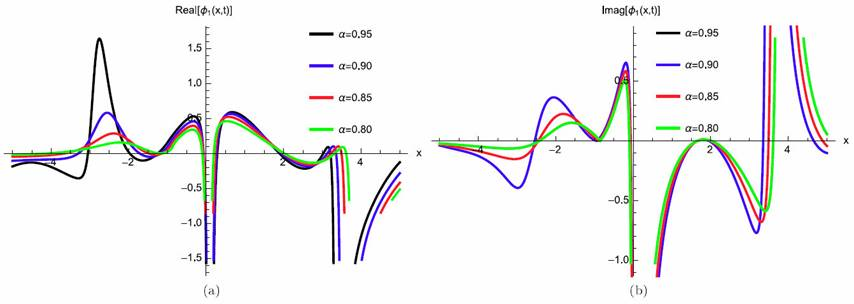Figure 2 2D Plot soliton solution for Eq. (25) for different particular cases of ρ, arbitrarily chosen.

[Set 2:] We obtain p = [i, -i, 1, 1] and q = [i, -i, i, -i], which gives

Λτ=-sinτcosτ. (26)

We also get

κ=-2θ-3λa-3bγ6γλ+θ, (27)

ν=(-9b2(-48λ2(-96λθ-48θ2γ2(+-12ρλ2+-6ab-24ρθλ-12ρθ2γ+4θ+3/2λθ+λ/2a212γ(λ+θ)2, (28)

ω=1216γ2λ+θ327bb2+48λ2+96λθ+48θ2γ3+432aλ3+864aθ+108ρbλ2+27ab2+432aθ2+216ρbθλ+108bρθ2γ2-36θ+3/2λa-2ρλ2+1/2ab-4ρθλ+abθ-2ρθ2γ-16(θ+3/2λ)23/4λ+θa3-216γ2λ+θ3, (29)

and

A0=0,A1=-6γ3λ+2θ,B1=-6γ3λ+2θ. (30)

Substituting above values into Eqs. (18) and (26), we have

Φτ=6γ3λ+2θ1cosτsinτ. (31)

Therefore an exact solution of Eq. (9) is obtained as

ϕ2x,t=6γ3λ+2θ1cos1αx+1Γαα-ναt+1Γααsin1αx+1Γαα-ναt+1Γαα×ei-καx+1Γαα+ωαt+1Γαα, (32)

where γ(3 + 2 θ) > 0 for valid soliton. Figures 3a)-3d) and 4a)-4b) show the soliton surface and the 2D graph for Eq. (32) respectively.Figure 3 3D soliton solution for Eq. (32) for different particular cases of ρ, arbitrarily chosen.Figure 4 2D Plot soliton solution for Eq. (32) for different particular cases of ρ, arbitrarily chosen.

[Set 3:] We obtain p = [1 - i, 1 + i, 1, 1] and q = [i, -i, i, -i], which gives

Λτ=cosτ+sinτcosτ. (33)

We also get

κ=-2θ-3λa-3bγ6γλ+θ, (34)

ν=-9b2+24λ2+48λθ+24θ2γ2+-12ρλ2+-6ab-24ρθλ-12ρθ2γ+4θ+3/2λθ+λ/2a212γλ+θ2, (35)

ω=1216γ2λ+θ327bb2-24λ2-48λθ-24θ2γ3+-216aλ3+-432aθ+108ρbλ2+27ab2-216aθ2+216ρbθλ+108bρθ2γ2-36(θ+3/2λ)a-2ρλ2+1/2ab-4ρθλ+abθ-2ρθ2γ-16θ+3/2λ23/4λ+θa3, (36)

and

A0=6γ2θ+3λ,A1=0,B1=-26γ2θ+3λ. (37)

Substituting above values into Eqs. (18) and (33), we have

Φτ=6γ2θ+3λsinτ-cosτ(cosτ+sinτ). (38)

Therefore an exact solution of Eq. (9) is obtained as

ϕ3x,t=6γ2θ+3λsin1αx+1Γαα-ναt+1Γαα-cos1αx+1Γαα-ναt+1Γααcos1αx+1Γαα-ναt+1Γαα+sin1αx+1Γαα-ναt+1Γαα×ei-καx+1Γαα+ωαt+1Γαα, (39)

where γ (3 λ + 2 θ) > 0 for valid soliton. Figures 5a)-5d) and 6a)-6b) show the soliton surface and the 2D graph for Eq. (39), respectively.Figure 5 3D soliton solution for Eq. (39) for different particular cases of ρ, arbitrarily chosen.Figure 6 2D Plot soliton solution for Eq. (39) for different particular cases of ρ, arbitrarily chosen.

[Set 4:]

We obtain p = [-1, 0, 1, 1] and q = [0, 0, 1, 0], which gives

Λ(τ)=-11+eτ. (40)

We also get

κ=-2θ-3λa-3bγ6γλ+θ, (41)

ν=-9b2-6λ2-12λθ-6θ2γ2+-12ρλ2+-6ab-24ρθλ-12ρθ2γ+4θ+3/2λθ+λ/2a212γλ+θ2, (42)

ω=1216γ2λ+θ327bb2+6λ2+12λθ+6θ2γ3+54aλ3+108aθ+108ρbλ2+27ab2+54aθ2+216ρbθλ+108bρθ2γ2-36θ+3/2λa-2ρλ2+1/2ab-4ρθλ+abθ-2ρθ2γ-16θ+3/2λ234λ+θa3, (43)

and

A0=126γ2θ+3λ,A1=6γ2θ+3λ,B1=0. (44)

Substituting above values into Eqs. (18) and (40), we have

Φ(τ)=126γ2θ+3λ6γeτ-12θ+3λeτ+1. (45)

Therefore an exact solution of Eq. (9) is obtained as

ϕ4x,t=126γ2θ+3λe1αx+1Γαα-ναt+1Γαα-1e1αx+1Γαα-ναt+1Γαα+1×ei-καx+1Γαα+ωαt+1Γαα. (46)

where γ (3 λ + 2 θ) > 0 for valid soliton. Figures 7a)-7d) and 8a)-8b) show the periodic wave surface and the 2D graph for Eq. (46), respectively.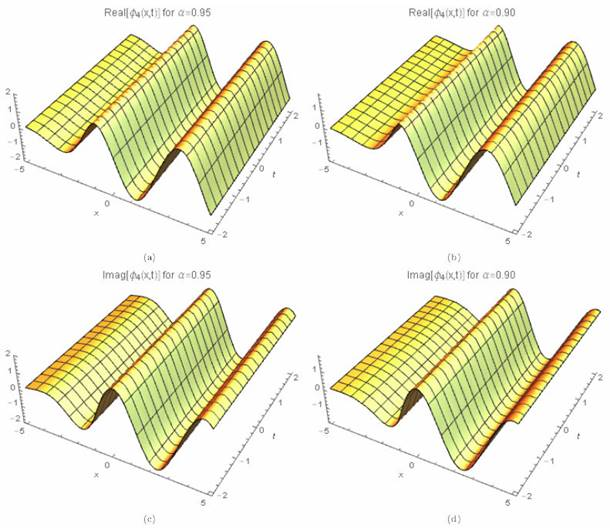Figure 7 3D periodic wave solution for Eq. (46) for different particular cases of ρ, arbitrarily chosen.Figure 8 2D Plot periodic wave solution for Eq. (46) for different particular cases of ρ, arbitrarily chosen.

[Set 5:] We obtain p = [-3, -1, -1, 1] and q = [-1, 1, -1, 1], which gives

Λτ=coshτ-2sinhτsinhτ. (47)

We also get

κ=-2θ-3λa-3bγ6γλ+θ, (48)

ν=(-9b2-24λ2-48λθ-24θ2)γ2+(-12ρλ2+(-6ab-24ρθ)λ-12ρθ2)γ+4(θ+3/2λ)(θ+λ/2)a212γλ+θ2, (49)

ω=1216γ2λ+θ327bb2+24λ2+48λθ+24θ2γ3+216aλ3+432aθ+108ρbλ2+27ab2+216aθ2+216ρbθλ+108bρθ2γ2-36θ+3/2λa-2ρλ2+1/2ab-4ρθλ+abθ-2ρθ2γ-16θ+3/2λ23/4λ+θa3-216γ2λ+θ3, (50)

and

A0=-26γ2θ+3λ,A1=0,B1=-36γ2θ+3λ. (51)

Substituting above values into Eqs. (18) and (47), we have

Φ(τ)=6γ2θ+3λ(sinhτ-2coshτ)(coshτ-2sinhτ). (52)

Therefore an exact solution of Eq. (9) is obtained as

ϕ5x,t=6γ2θ+3λsinh1αx+1Γαα-ναt+1Γαα-2cosh1αx+1Γαα-ναt+1Γααcosh1αx+1Γαα-ναt+1Γαα-2sinh1αx+1Γαα-ναt+1Γαα×ei-καx+1Γαα+ωαt+1Γαα, (53)

where γ (3 λ + 2 θ) > 0 for valid soliton. Figures 9a)-9d) and 10a)-10b) show the periodic wave surface and the 2D graph for Eq. (53), respectively.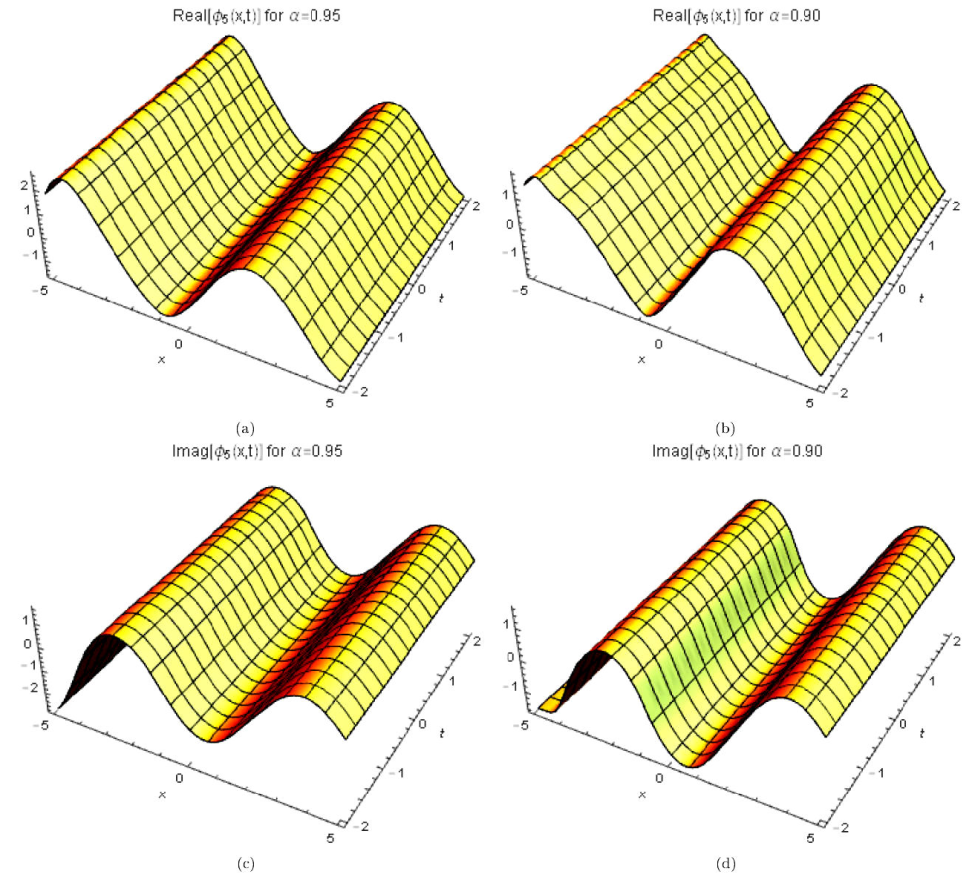Figure 9 3D periodic wave solution for Eq. (53) for different particular cases of ρ, arbitrarily chosen.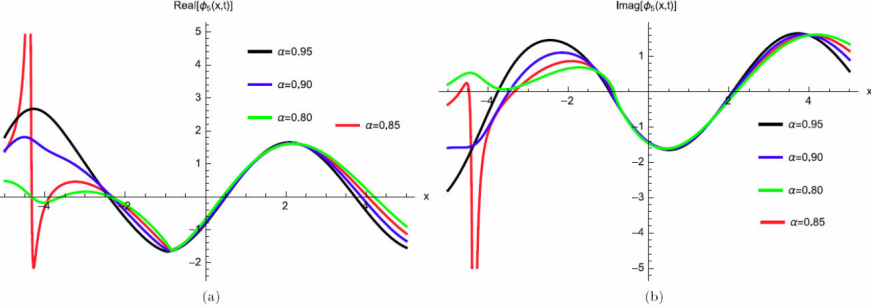Figure 10 2D Plot periodic wave solution for Eq. (53) for different particular cases of ρ, arbitrarily chosen.

[Set 6:] We obtain p = [1 - i, 1 + i, 1, 1] and q = [-i, i, -i, i], which gives

Λτ=cosτ-sinτcosτ. (54)

We also get

κ=(-2θ-3λ)a-3bγ6γ(λ+θ), (55)

ν=-9b2-96λ2-192λθ-96θ2γ2+-12ρλ2+-6ab-24ρθλ-12ρθ2γ+4θ+3/2λθ+λ/2a212γλ+θ2, (56)

ω=1216γ2λ+θ327bb2+96λ2+192λθ+96θ2γ3+864aλ3+1728aθ+108ρbλ2+27ab2+864aθ2+216ρbθλ+108bρθ2γ2-36θ+3/2λa-2ρλ2+1/2ab-4ρθλ+abθ-2ρθ2γ-16θ+3/2λ23/4λ+θa3, (57)

and

A0=0,A1=-6γ2θ+3λ,B1=6γ2θ+3λ. (58)

Substituting above values into Eqs. (18) and (54), we have

Φ(τ)=6γ2θ+3λcoth2(τ)+1coth(τ). (59)

Therefore an exact solution of Eq. (9) is obtained as

ϕ6x,t=6γ2θ+3λcoth21αx+1Γαα-ναt+1Γαα+1coth1αx+1Γαα-ναt+1Γααei-καx+1Γαα+ωαt+1Γαα. (60)

where γ (3 λ + 2 θ) > 0 for valid soliton. Figures 11a)-11d) and 12a)-12b) show the periodic wave surface and the 2D graph for Eq. (60), respectively.Figure 11 3D periodic wave solution for Eq. (60) for different particular cases of ρ, arbitrarily chosen.Figure 12 2D Plot periodic wave solution for Eq. (60) for different particular cases of ρ, arbitrarily chosen.

[Set 7:] We obtain p = [2, 0, 1, -1] and q = [1, 0, 1, -1], which gives

Λτ=coshτ+sinhτsinhτ. (61)

We also get

κ=-2θ-3λa-3bγ6γλ+θ, (62)

ν=-9b2-24λ2-48λθ-24θ2γ2+-12ρλ2+-6ab-24ρθλ-12ρθ2γ+4θ+3/2λθ+λ/2a212γλ+θ2, (63)

ω=1216γ2λ+θ327bb2+24λ2+48λθ+24θ2γ3+216aλ3+432aθ+108ρbλ2+27ab2+216aθ2+216ρbθλ+108bρθ2γ2-36θ+3/2λa-2ρλ2+1/2ab-4ρθλ+abθ-2ρθ2γ-16θ+3/2λ23/4λ+θa3, (64)

and

A0=-6γ2θ+3λ,A1=6γ2θ+3λ,B1=0. (65)

Substituting above values into Eqs. (18) and (61), we have

Φ(τ)=6γ2θ+3λcoshτsinhτ. (66)

Therefore an exact solution of Eq. (9) is obtained as

ϕ7x,t=6γ2θ+3λcosh1αx+1Γαα-ναt+1Γααsinh1αx+1Γαα-ναt+1Γααei-καx+1Γαα+ωαt+1Γαα, (67)

where γ (3 λ + 2 θ) > 0 for valid soliton. Figures 13a)-13d) and 14a)-14b) show the soliton surface and the 2D graph for Eq. (67), respectively.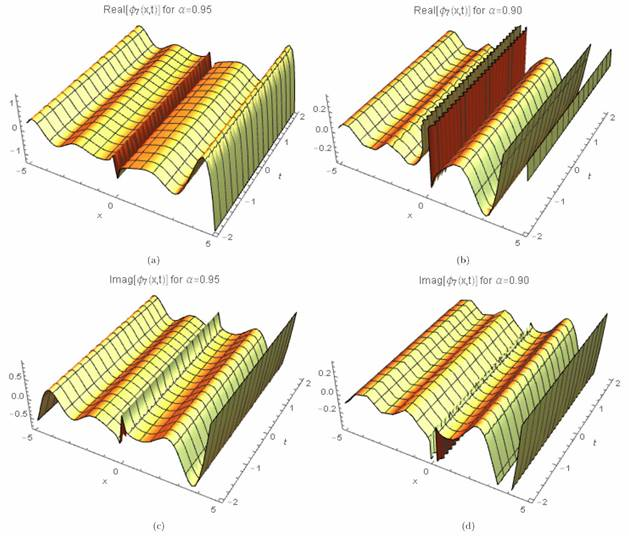Figure 13 3D soliton surface for Eq. (67) for different particular cases of ρ, arbitrarily chosen.Figure 14 2D Plot soliton surface for Eq. (67) for different particular cases of ρ, arbitrarily chosen.

4. Results and discussion

We discuss the results to Eq. (9) obtained by using the generalized exponential rational function method. As a result, we get new form of solitary traveling wave solutions for this model including novel soliton, traveling waves and kink-type solutions with complex structures. In this work, by using the GERFM, we successfully constructed some exponential and rational function solutions to Eq. (9). When we compare our results with the results reported in [40-47], we observed that, all the results obtained in this study by using the above method are newly structured solutions. These soliton solutions are located throughout parameter restrictions that provide their existence and novel soliton, traveling waves and kink-type solutions with complex structures while other solutions that emerged from the Laplace-Adomian decomposition method, traveling wave hypothesis, extended trial function scheme, among others. These soliton solutions are being reported for the first time in this paper. It shows that the GERFM give an effective and credible mathematical tool for various nonlinear evolution equations to obtain a variety of wave solutions. All solutions are satisfied with their corresponding original equations.

5. Conclusion

In this work, we have obtain exact analytical solutions including novel soliton, traveling waves and kink-type solutions with complex structures for the perturbed β-conformable-time Radhakrishnan-Kundu-Lakshmanan equation. The generalized exponential rational function method is successfully applied to obtain soliton solutions. It is worth mentioning that whole solutions of φ 1 - φ 9 in this article are new and being reported for the first time. From our results obtained in this paper, we conclude that the generalized exponential rational function method is powerful, effective and convenient for the analysis of nonlinear conformable-time partial differential equations involving β-conformable derivative. The obtained results may be utilized to understand the certain physical phenomenons in science and engineering in general and in fiber optics in particular.

Acknowledgments

José Francisco Gómez Aguilar acknowledges the support provided by CONACyT: Cátedras CONACyT para jóvenes investigadores 2014 and SNI-CONACyT.

References

1. X.J. Yang, F. Gao, and H.M. Srivastava, Computers & Mathematics with Applications 73 (2017) 203-210. [ Links ]

2. O.S. Iyiola, E.O. Asante-Asamani, and B.A. Wade, Journal of Computational and Applied Mathematics 330 (2018) 307-317. [ Links ]

3. Y. Bai, D. Baleanu and G.C. Wu, Communications in Nonlinear Science and Numerical Simulation 59 (2018) 338-348. [ Links ]

4. R. Garrappa and M. Popolizio, Journal of Scientific Computing 1 (2018) 1-25. [ Links ]

5. Y. Mousavi and A. Alfi, Chaos, Solitons & Fractals, 114 (2018) 202-215. [ Links ]

6. A. Fernandez, D. Baleanu, and H.M. Srivastava , Communications in Nonlinear Science and Numerical Simulation, 67 (2018) 517-527. [ Links ]

7. C.S. Liu, Chaos, Solitons & Fractals 109 (2018) 219-222. [ Links ]

8. I. Podlubny, Fractional Differential Equations: An Introduction to Fractional Derivatives, Fractional Differential Equations, to Methods of Their Solution and some of Their Applications. Academic Press, (San Diego, Calif, USA, 1999). [ Links ]

9. A. Atangana and D. Baleanu., Theory and Application to Heat Transfer Model. Therm Sci. 20 (2016) 763-769. [ Links ]

10. M. Caputo and M. Fabricio, Progr. Fract. Differ. Appl. 1 (2015), 73-85. [ Links ]

11. H. Yépez-Martínez and J.F. Gómez-Aguilar, Waves in Random and Complex Media, 1 (2018) 1-16. [ Links ]

12. H. Yépez-Martínez, J.F. Gómez-Aguilar andD. Baleanu, Optik-International Journal for Light and Electron Optics 155 (2018) 357-365. [ Links ]

13. M. Ahmad, A.A. Bhat and R. Jain, Journal of New Theory (22) (2018) 73-81. [ Links ]

14. A. Alizadeh and S. Effati, Transactions of the Institute of Measurement and Control 40 (2018) 2054-2061. [ Links ]

15. D. Rani and V. Mishra, European Journal of Pure and Applied Mathematics, 11 (2018) 202-214. [ Links ]

16. D. Kumar, R.P. Agarwal and J. Singh, Journal of Computational and Applied Mathematics 339 (2018) 405-413. [ Links ]

17. B.K. Singh, P. Kumar and V. Kumar, International Journal of Applied and Computational Mathematics, 4 (2018) 1-19. [ Links ]

18. N. Das, R. Singh, A.M. Wazwaz, and J. Kumar, Journal of Mathematical Chemistry 54 (2016) 527-551. [ Links ]

19. G.C. Wu, D. Baleanu, and Z.G. Deng, Applied Mathematical Modelling 39 (2015) 4378-4384. [ Links ]

20. G.C. Wu andD. Baleanu, Applied Mathematical Modelling, 37 (2013) 6183-6190. [ Links ]

21. J.S. Duan, R. Rach and A.M. Wazwaz , International Journal of Computer Mathematics 1 (2015) 1-19. [ Links ]

22. D. Kumar, J. Singh and S. Kumar, Walailak Journal of Science and Technology (WJST) 11 (2013) 711-728. [ Links ]

23. D. Kumar , J. Singh and S. Kumar, Journal of the Egyptian Mathematical Society, 22 (2014) 373-378. [ Links ]

24. R. Khalil, M. Al Horani, A. Yousef, and M. Sababheh, Journal of Computational and Applied Mathematics, 264 (2014) 65-70. [ Links ]

25. A. Yusuf, A.I. Aliyu andD. Baleanu, Optical and Quantum Electronics, 50 (2018) 1-20. [ Links ]

26. A. Akbulut, and M. Kaplan, Computers & Mathematics with Applications, 75 (2018) 876-882. [ Links ]

27. H.W. Zhou, S. Yang, and S.Q. Zhang, Physica A: Statistical Mechanics and its Applications, 491 (2018) 1001-1013. [ Links ]

28. C. Chen and Y.L. Jiang, Computers & Mathematics with Applications, 75 (2018) 2978-2988. [ Links ]

29. O. Acan, O. Firat and Y. Keskin, Waves in Random and Complex Media 1 (2018) 1-19. [ Links ]

30. A. Korkmaz, Communications, 67 (2018) 68-79. [ Links ]

31. M.T. Darvishi, M. Najafi and A.R. Seadawy, Optical and Quantum Electronics, 50 (2018) 1-18. [ Links ]

32. B. Abdalla, Advances in Difference Equations, 2018 (2018) 1-17. [ Links ]

33. O. Ozkan, and A. Kurt, Optical and Quantum Electronics, 50 (2018) 1-18. [ Links ]

34. A. Yusuf, A.I. Aliyu, andD. Baleanu, Optik 162 (2018) 65-75. [ Links ]

35. A. Atangana, Neural Comput. Appl. 26 (2015) 1895-1903. [ Links ]

36. E. Bonyah, A. Atangana and M.A. Khan, Asia Pacific Journal on Computational Engineering, 4 (2017) 1-18. [ Links ]

37. A. Atangana and C. Ünlü, Scientia Iranica. Trans. B Mech. Eng. 23 (2016) 1837-1843. [ Links ]

38. A. Atangana, D. Baleanu, and A. Alsaedi, Open Phys. 14 (2016) 145-149. [ Links ]

39. A. Atangana, and B.S.T. Alkahtani, Complexity 21 (2016) 442-451. [ Links ]

40. A Biswas, Physics Letters A 373 (2009) 2546-2548. [ Links ]

41. B. Sturdevant, D.A. Lott, and A. Biswas, Mod. Phys. Lett. B 24 (2010) 1825-1831. [ Links ]

42. D. Lu, A. R. Seadawy and M.M.A. Khater, Optik 164 (2018) 54-64. [ Links ]

43. A. Biswas, M. Ekici, A. Sonmezoglu and A. Alshomrani, Optik 160 (2018) 415-427. [ Links ]

44. A. Biswas et al., Optik, 163 (2018) 126-136. [ Links ]

45. A. Biswas, Optik 171 (2018) 217-220. [ Links ]

46. A. Bansal et al., Optik 163 (2018) 137-141. [ Links ]

47. T.A. Sulaiman, H. Bulut, G. Yel and S.S. Atas, Optical and Quantum Electronics 50 (2018) 1-8. [ Links ]

48. B. Ghanbari and M. Inc, Eur. Phys. J. Plus 133 (2018) 1-16. [ Links ]

49. M.S. Osmana and B. Ghanbari , Optik, 175 (2018) 328-333. [ Links ]

Received: January 14, 2019; Accepted: February 08, 2019

* E-mail: jgomez@cenidet.edu.mx.

Competing interests. The authors declare that there is no conflict of interests regarding the publication of this paper.

Authors’ contributions. All authors contributed equally and significantly in writing this article. All authors read and approved the final manuscript.This is an open-access article distributed under the terms of the Creative Commons Attribution License